「APIO2010」巡逻 - 动规/贪心 | Bill Yang's Blog

# 路终会有尽头，但视野总能看到更远的地方。

0%

## 方法1：贪心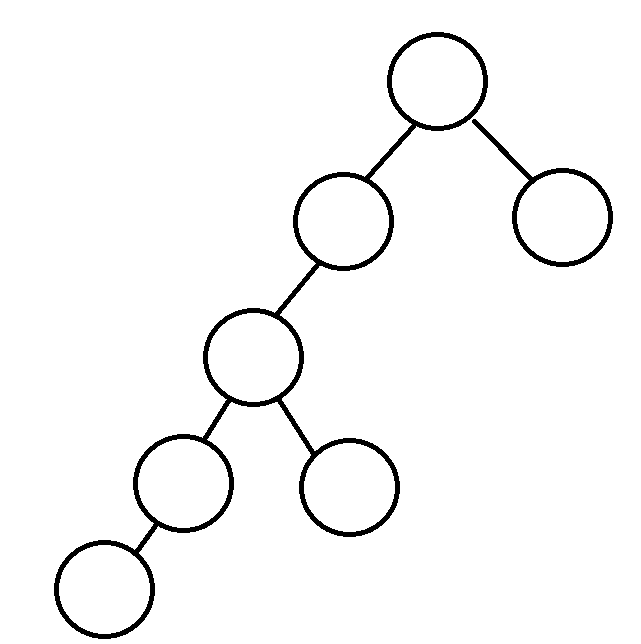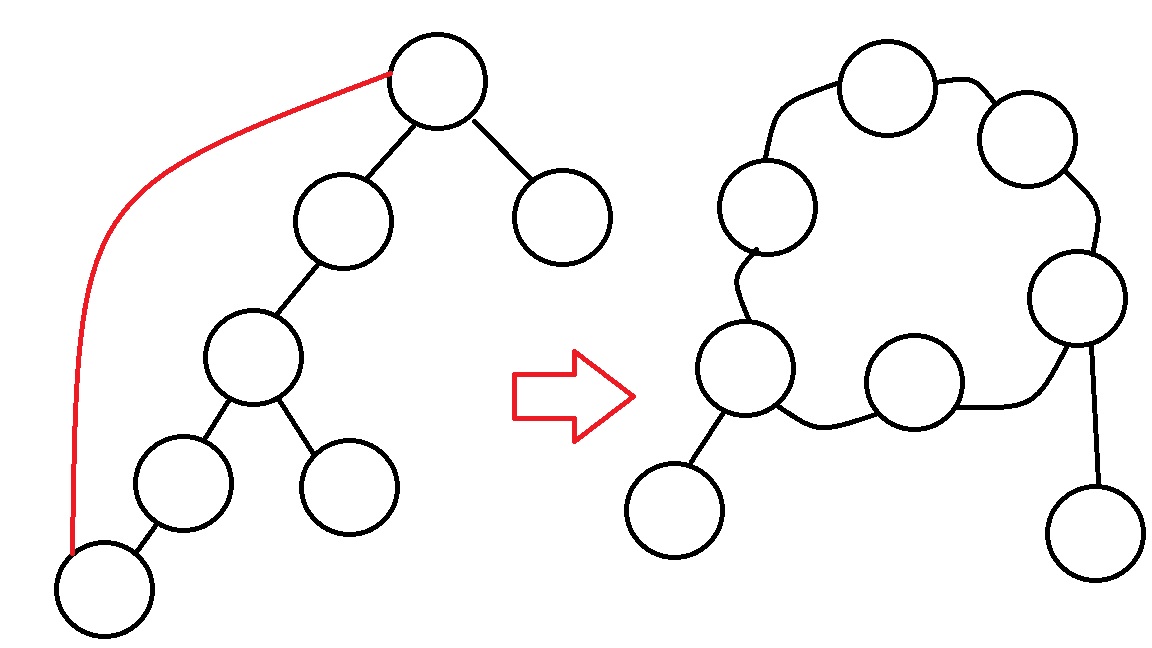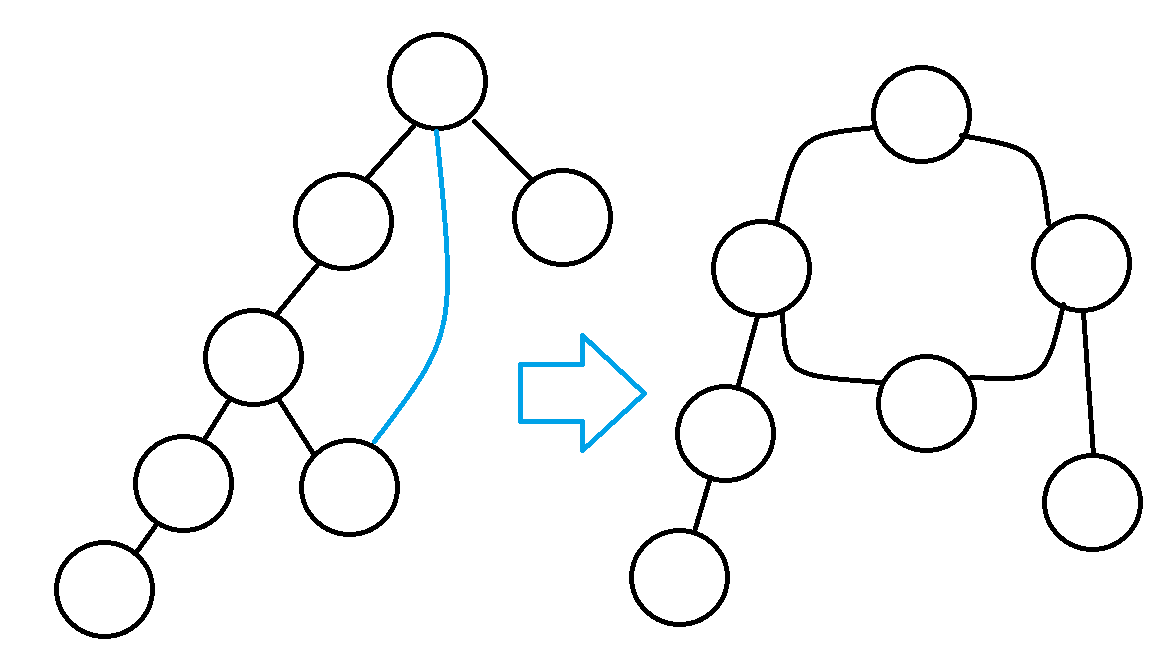$\quad$①环越大越好
$\quad$②新环与原环相交部分越少越好

## 方法2：树形动规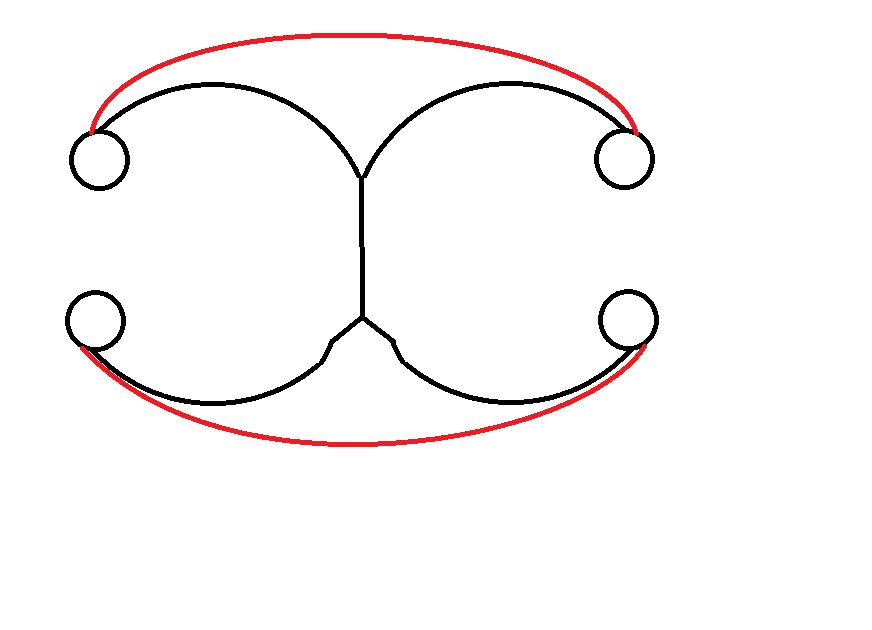• 情况1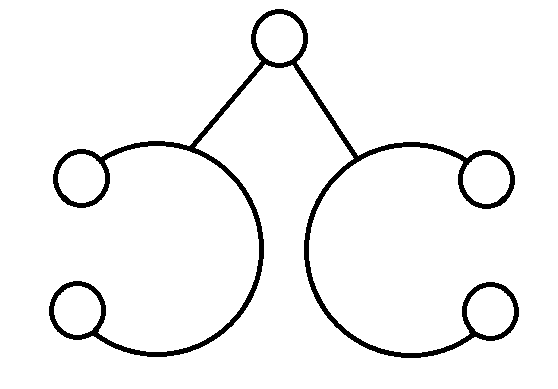如图，即当前结点的所有后代中有两个环链。（规定必须是后代，不包含自己）
环链的求法和直径一样，即为当前结点最长链+次长链。
维护最长链d1[]与次长链d2[]
易得当前结点子树中环链的最大长度：统计当前结点的子结点Circle[]的最大c1[]与次大c2[]，使用$c1[i]+c2[i]$更新答案
• 情况2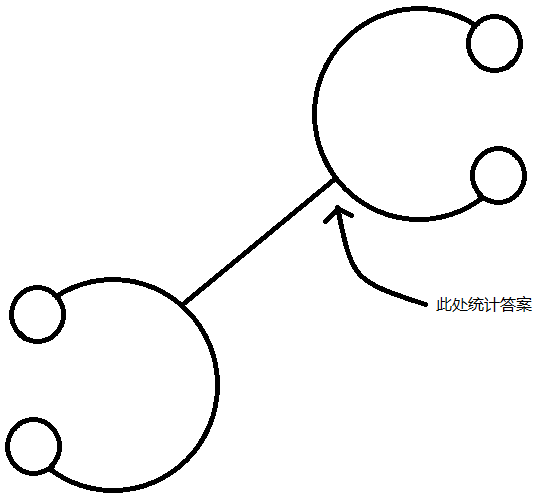如图，即其中一个环链是另一个环链的祖先。（规定必须严格祖先后代关系，不能相交）
根据上图，选择箭头所指点统计答案较为便捷。
当前已经有了第一个环链（后代），但是要构成第二个环链（祖先），需要讨论环链如何构成。（注：du表示来自父亲的最长链）
$\quad$ ①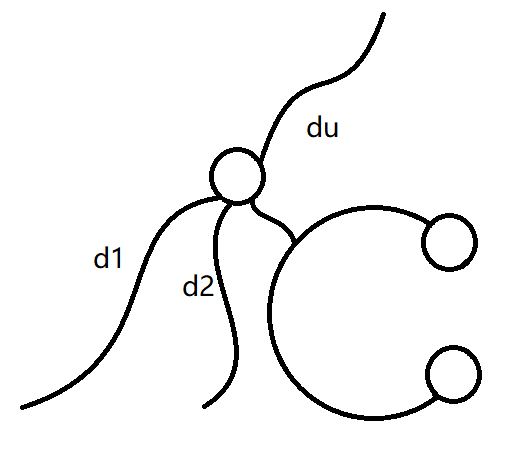$\quad$ 如图，最长链与次长链均不来自环链，du+d1与d1+d2可以构成两个环链
$\quad$ 使用$max(d2[Now],du[Now])+d1[Now]+Circle[Next]$更新答案
$\quad$
$\quad$ ②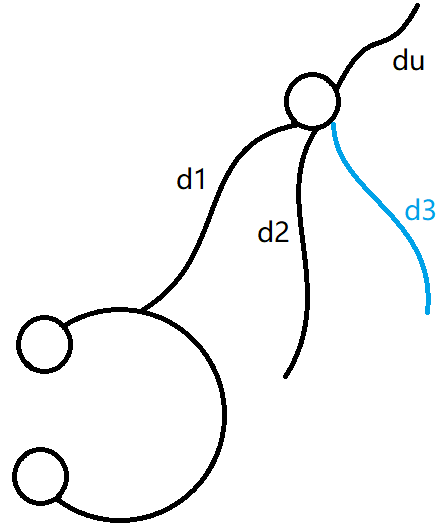$\quad$ 如图，最长链来自环链（判断方法：d1[Next]+1==d1[Now]），那么有了环链，就不能使用d1+d2更新答案。
$\quad$ 为了构成祖先环链，我们还需维护第三长链d3[]，那么du+d2与d2+d3可以构成两个环链
$\quad$ 使用$max(d3[Now],du[Now])+d2[Now]+Circle[Next]$更新答案
$\quad$
$\quad$ ⑨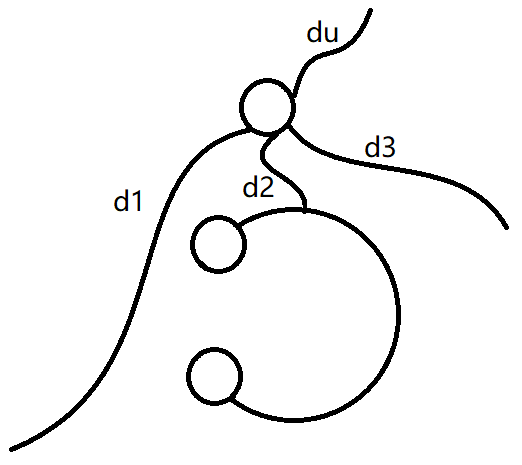$\quad$ 类似的，次长链来自环链（判断方法：d1[Next]+1==d2[Now]），不能使用d1+d2更新答案。
$\quad$ du+d2与d2+d3可以构成两个环链
$\quad$ 使用$max(d3[Now],du[Now])+d1[Now]+Circle[Next]$更新答案
$\quad$
那么情况2就讨论完了。
• 情况3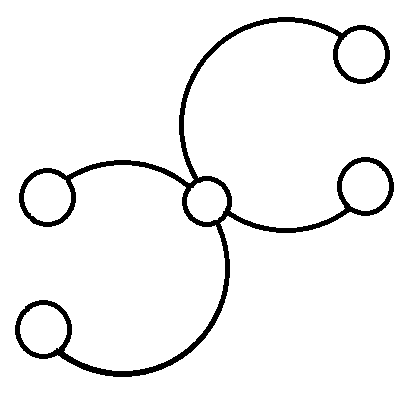如图，两个环链有交点。（边不相交，点相交）
在交点处统计答案较为便捷。
故得到以下图形：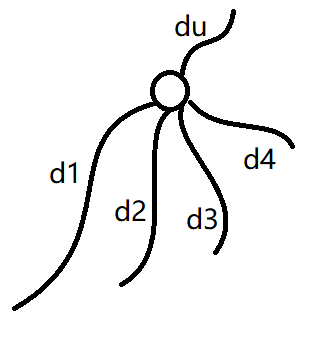由图，还需要维护第四长链d4[]
显然可以使用$d1[Now]+d2[Now]+d3[Now]+max(d4[Now],du[Now])$更新答案

• d1[]~d4[]：最长链~第四长链
• du[]：来自父亲的最长链
• Circle[]：当前结点子树中环链的最大长度
• c1[]、c2[]：当前结点儿子的Circle值最大与次大